# MINERvA Review

## Postdoc summary

University of Rochester

Department of Physics and Astronomy

MINERvA (prof. Kevin McFarland)

• flux systematic errors
• physics reconstruction using machine learning
• generator group coordinator

Fermi National Accelerator Laboratory

Scientific Computing Division

GENIE (dr. Gabe Perdue)

• nuclear effects in GENIE
• automated validation system
• "user support"

## MINERvA Experiment

• MINERvA is a neutrino-scattering experiment at Fermilab
• Collaboration of about 50-100 physicist
• NuMI beam is used to measure cross section for neutrino-nucleus interactions
• The detector includes several different nuclear targets## Detector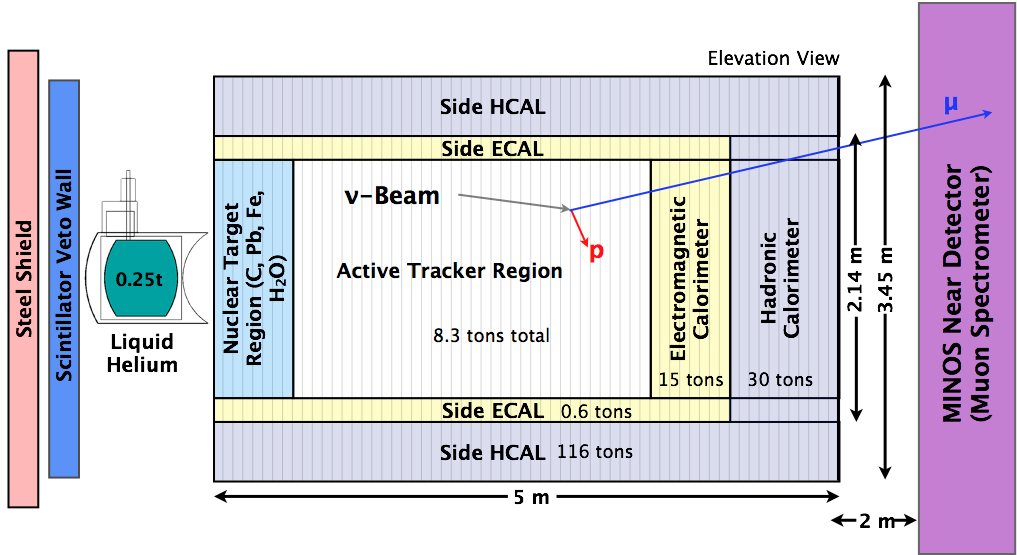## Nuclear targets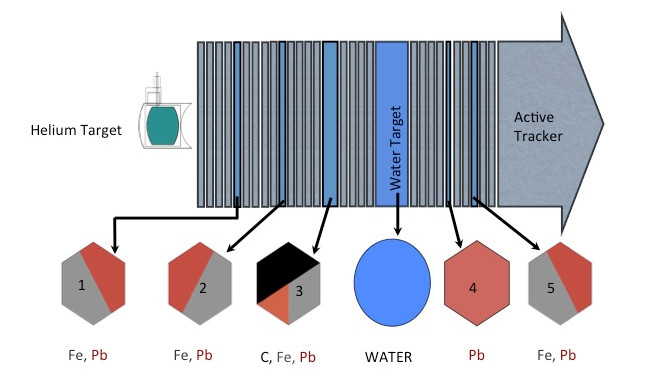## NuMI Beamline## Low vs Medium Energy

• by changing distance between horns one can change energy spectrum

• by changing horns polarization one can switch between neutrino and anti-neutrino mode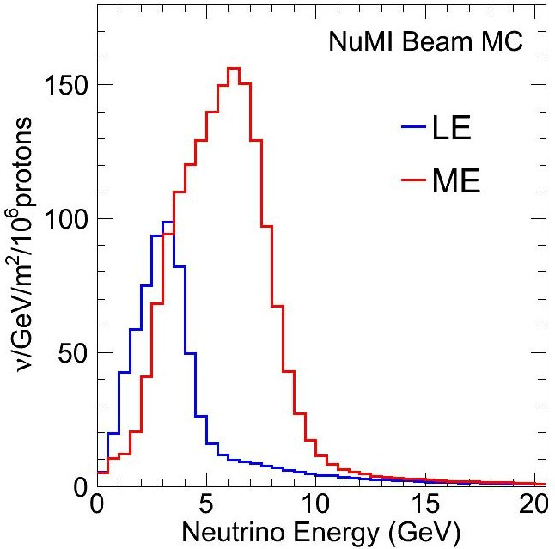## NuMI Beam Simulation• Flux simulation starts with a Geant4 with the NuMI geometry

• All of the information about interactions leading to neutrino are stored

• The results of the simulation are corrected by external data

• Similar approach to the one T2K used

• NA49 - charged hadron production in proton scattering off thin targets

• FLUKA is used to scale proton energy from $$158$$ to $$120$$ GeV

• MIPP - charged hadron production on thin target and NuMI target replica

## NA49 Data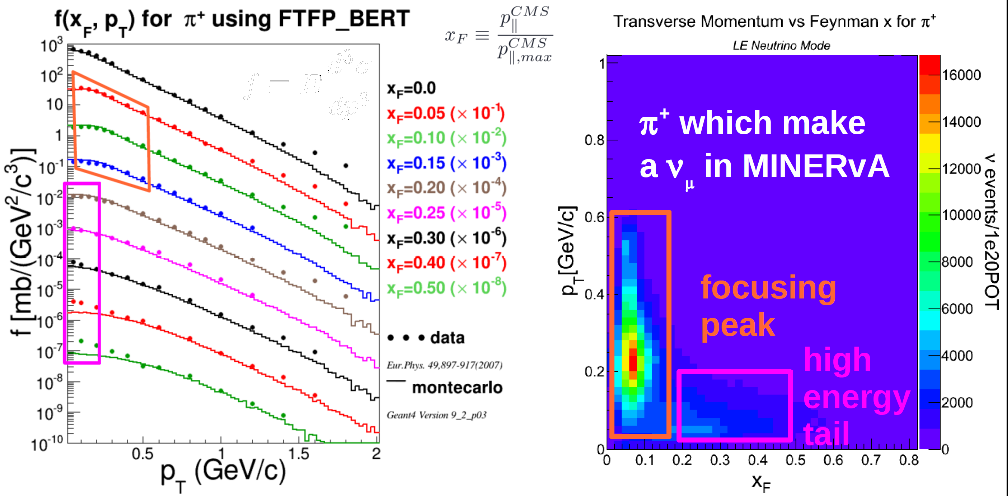## MIPP data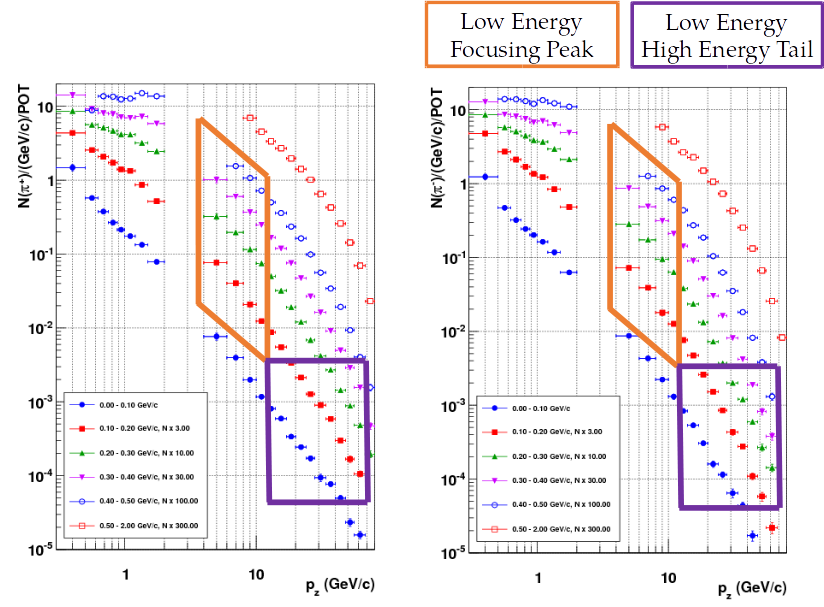## Reweighting

$w_{HP} = \frac{f_{data}(x_F, p_T, E)}{f_{MC}(x_F, p_T, E)}, ~~~~~ f\equiv\frac{E}{\sigma}\frac{d^3\sigma}{dp^3}$

• Using external hadron production data events are weighted using the above formula

• An event is reweighted on "interaction-by-interaction" basis

• Whenever possible - "thick" target data is used

## Uncertainties

• Many-Universes method is used to propagate external data uncertainties to our flux

• for each universe (u) data central value is shifted (respect to data uncertainties)

$w_u \sim \prod_i w_{HP, u, i}$## Flux generations

• Generation 0 -> no MIPP data

• Generation 1 -> MIPP thin target data + other improvements

• Generation 2 -> MIPP thick target data + other improvements

## Flux vs publications

Flux Analysis Reference
Generation 0 $$\nu_\mu$$ CCQE PRL 111 (2013) 022502
Generation 0 $$\bar{\nu_\mu}$$ CCQE PRL 111 (2013) 022501
Generation 1 $$\nu_\mu$$ muon + proton PRD 91 (2015) 071301
Generation 1 $$\nu_\mu$$ CC $$\pi^\pm$$ PRD 92 (2015) 092008
Generation 1 $$\bar{\nu_\mu}$$ CC $$\pi^0$$ PLB 749 (2015) 130
Generation 1 Coherent $$\pi$$ PRL 113 (2014) 261802
Generation 1 CC target ratios PRL 112 (2014) 231801

## Generation 0 vs Generation 2 (thick off)## Generation 1 vs Generation 2 (thick off)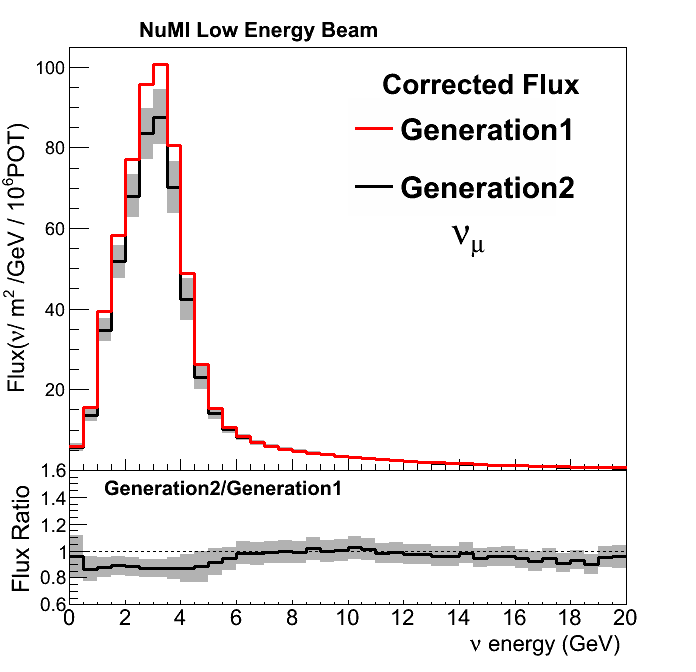## Generation 2 (thin vs thick)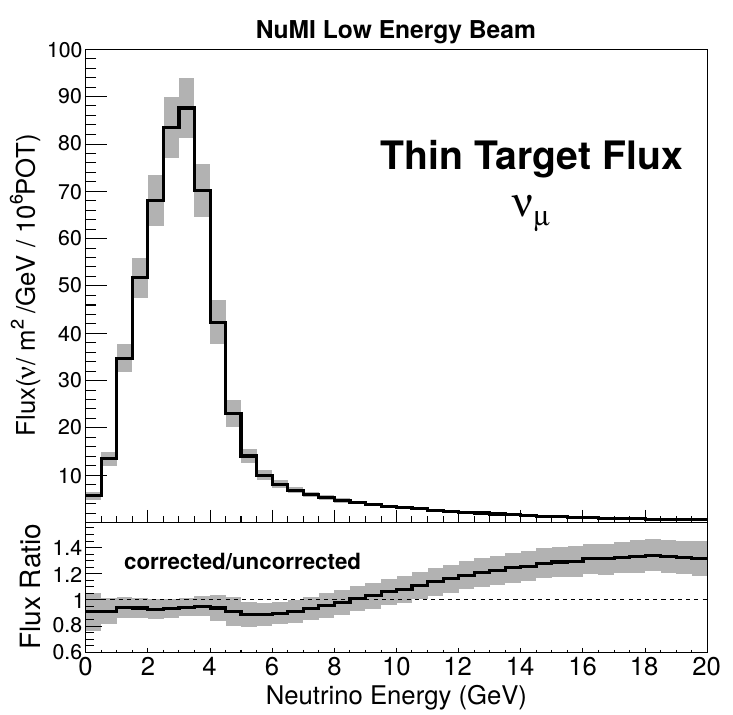## Generation 2: ratio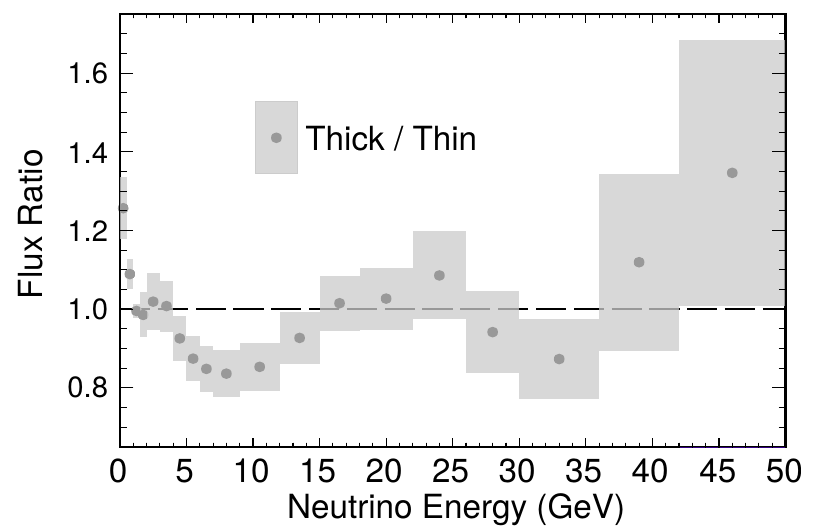## $$\nu e$$ constraint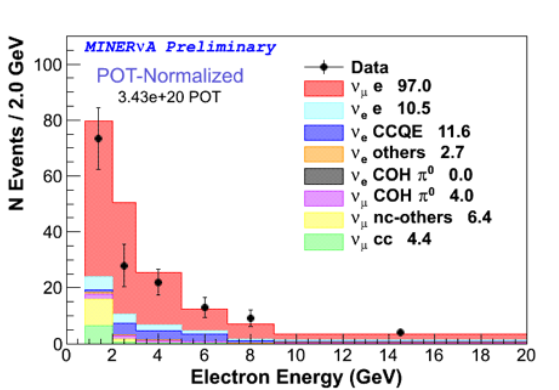• Weighting up universes that agree better with data
• Experimental signature is a very forward single electron in the finale state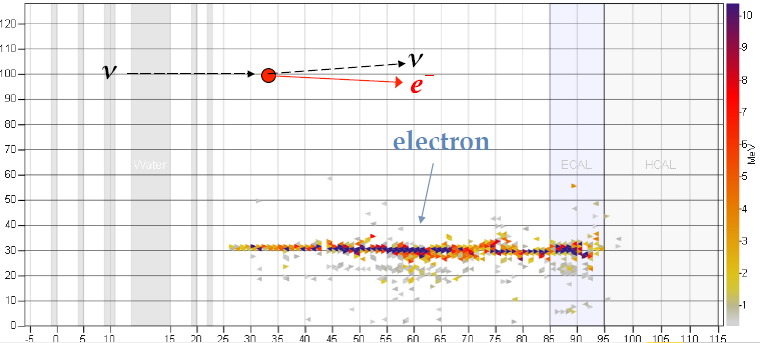## Generation 1 + $$\nu e$$## low-$$\nu$$ method

$\frac{d\sigma}{d\nu} = A + B\frac{\nu}{E} - \frac{C}{2}\frac{\nu^2}{E^2}$

• Differential cross-section can be expressed by the above formula

• It is a constant for $$\frac{\nu}{E} \rightarrow 0$$

• It can be used to constraint the flux prediction (with high-energy normalization taken from other measurements, like NOMAD)

## low-$$\nu$$ vs generation 2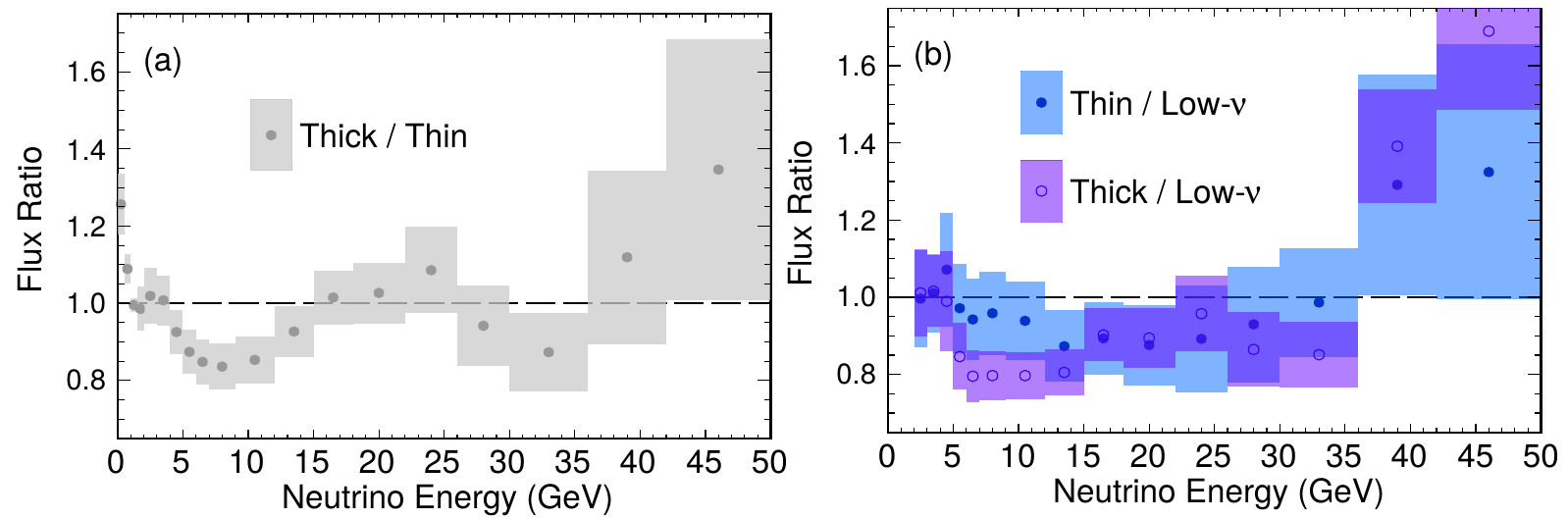## CCQE-"true" aka "1-track"

Flux Analysis Reference
Generation 0 $$\nu_\mu$$ CCQE PRL 111 (2013) 022502
Generation 0 $$\bar{\nu_\mu}$$ CCQE PRL 111 (2013) 022501

• require only muon track

• target -> scintillator (CH)

## High-$$Q^2$$ candidates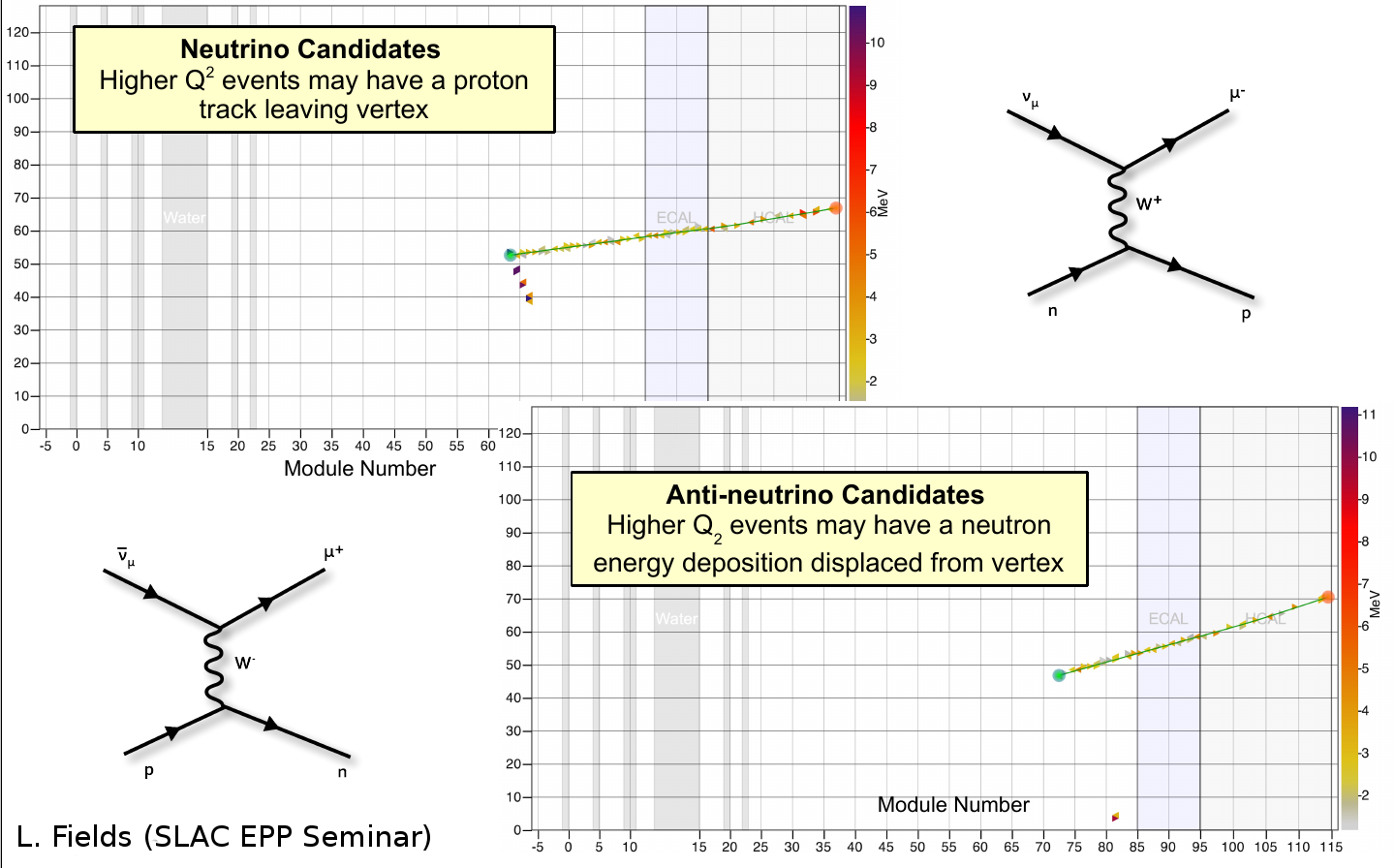## Low-$$Q^2$$ candidates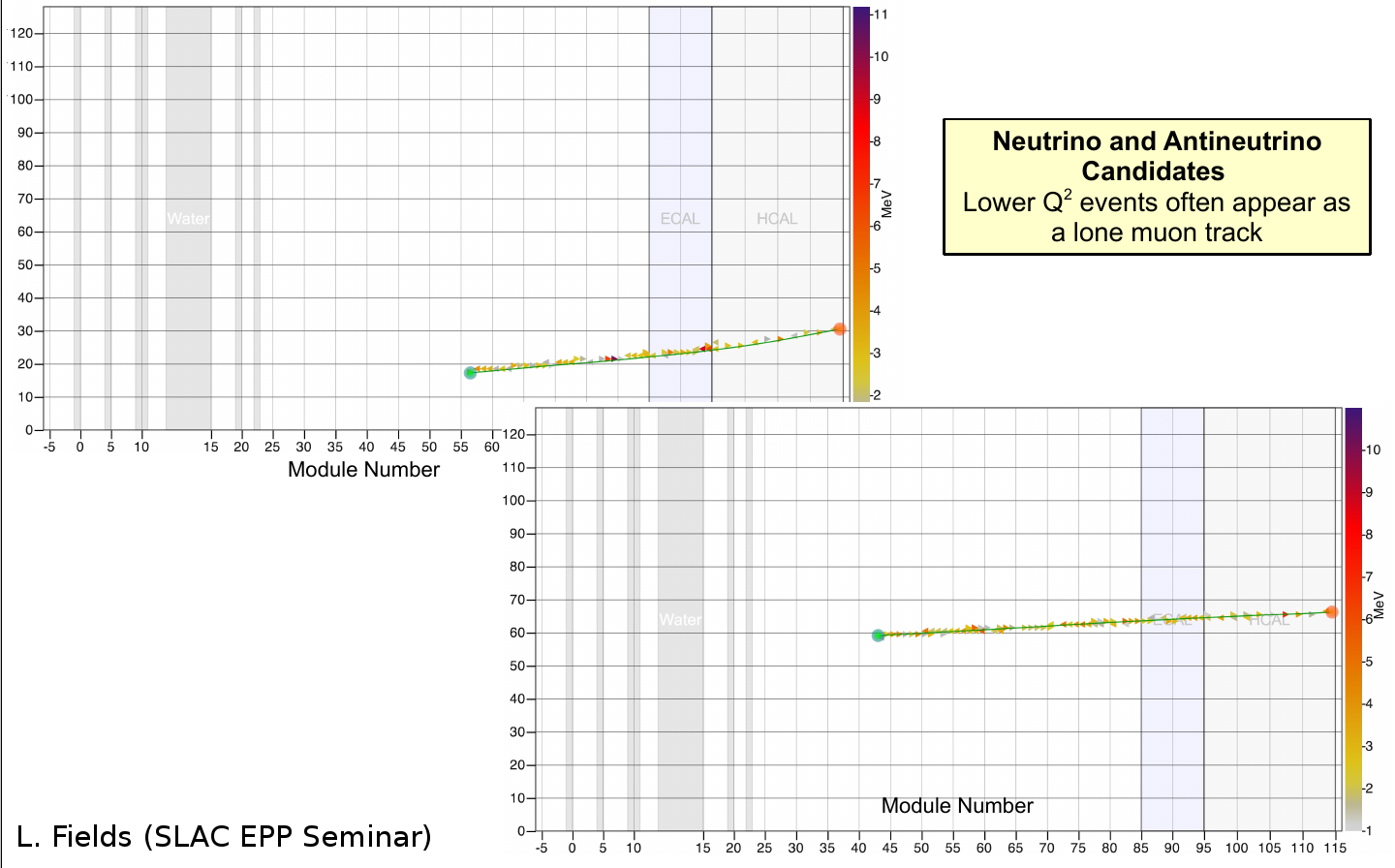## Background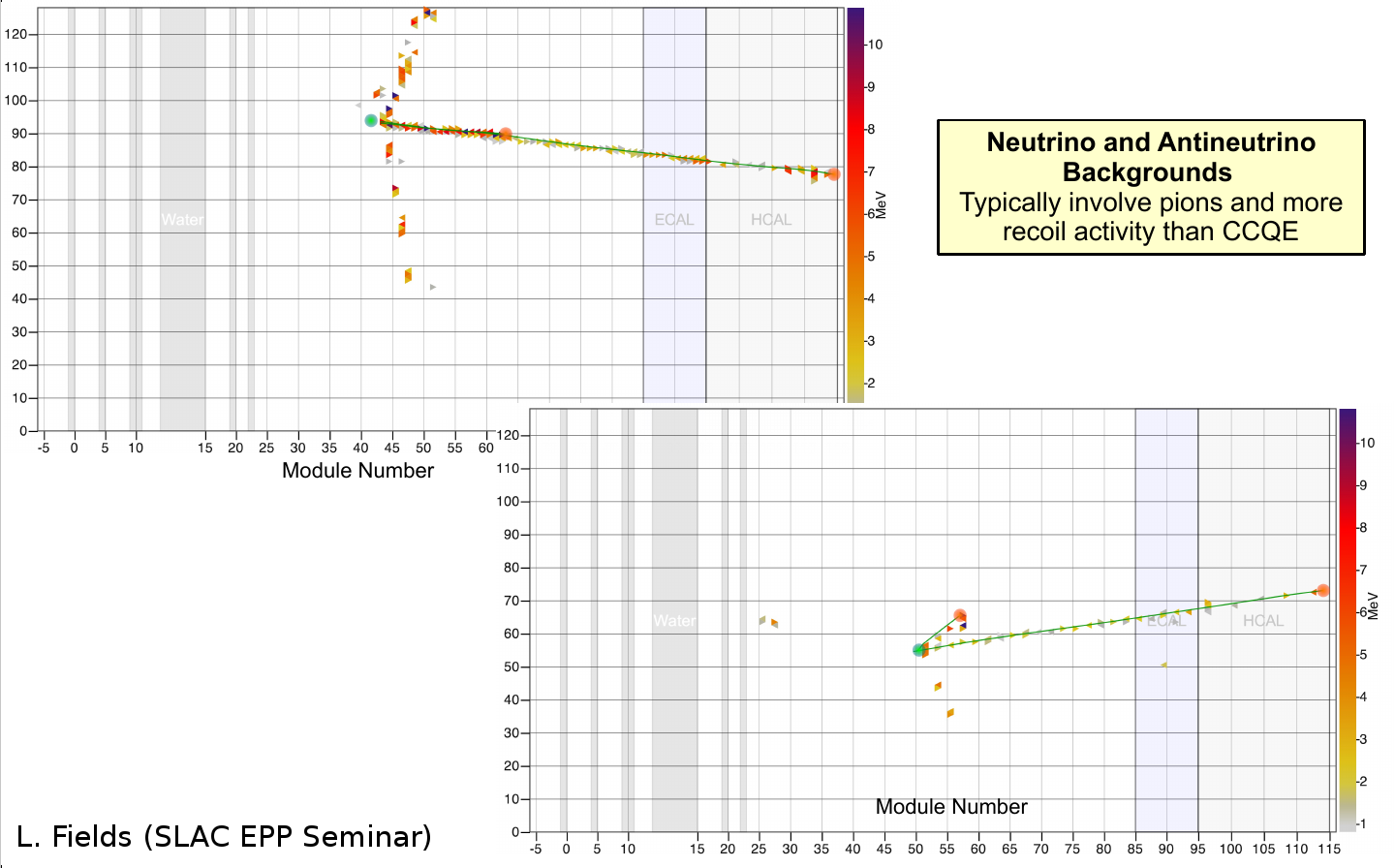## Background subtraction## $$\nu_\mu$$ CCQE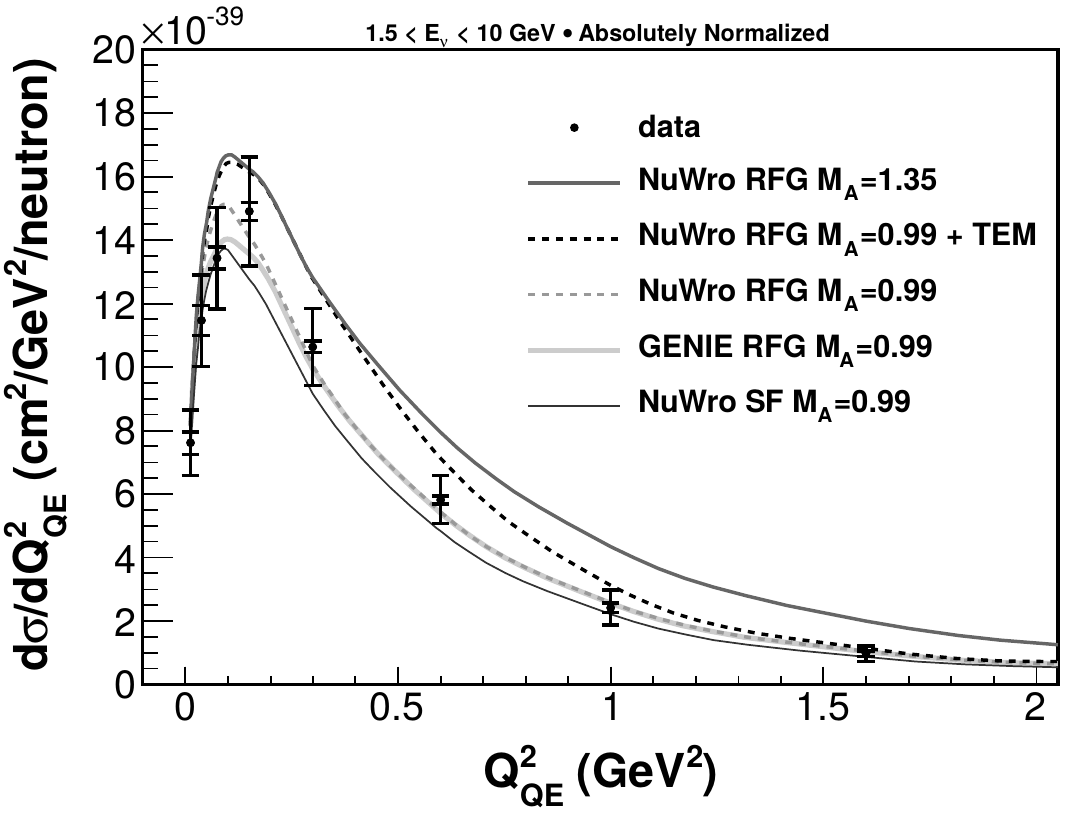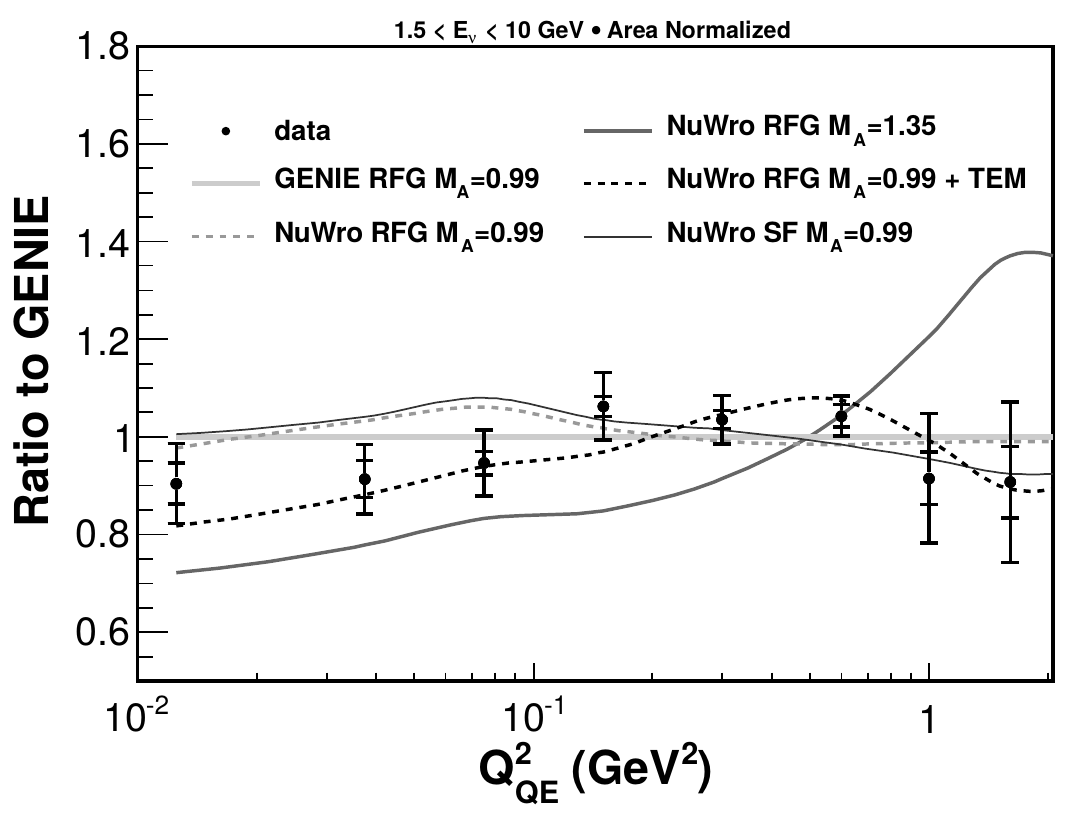## $$\bar\nu_\mu$$ CCQE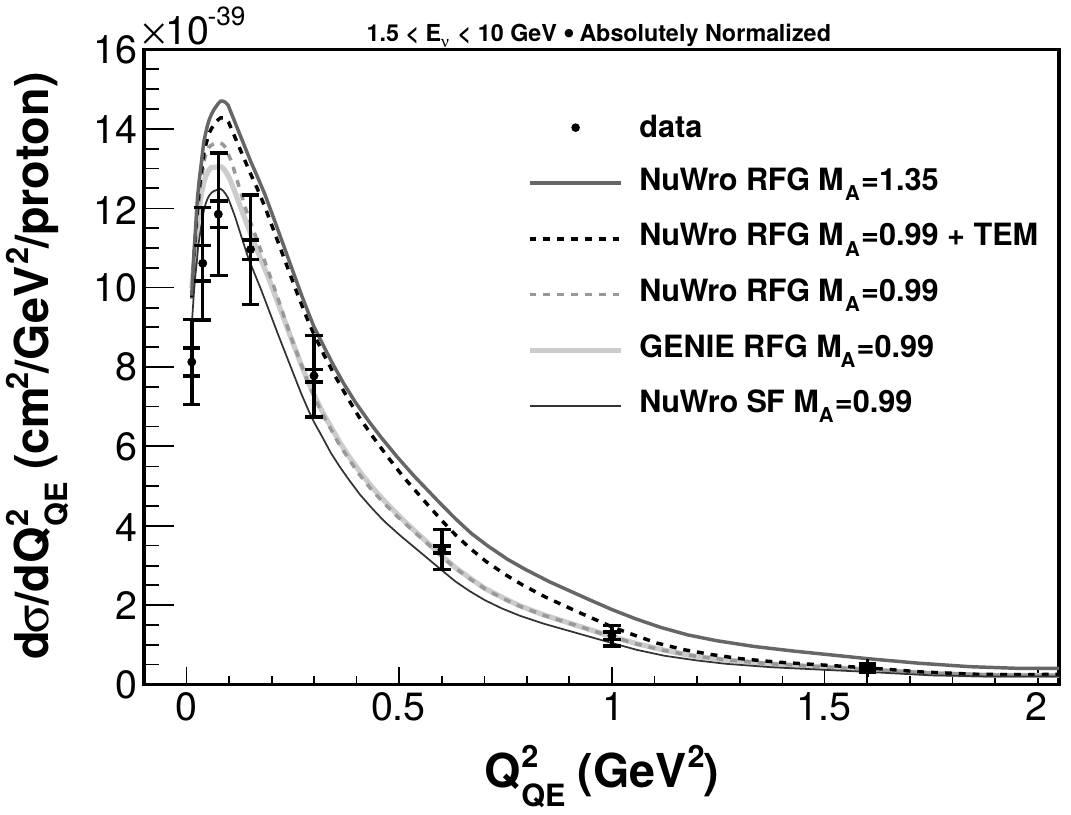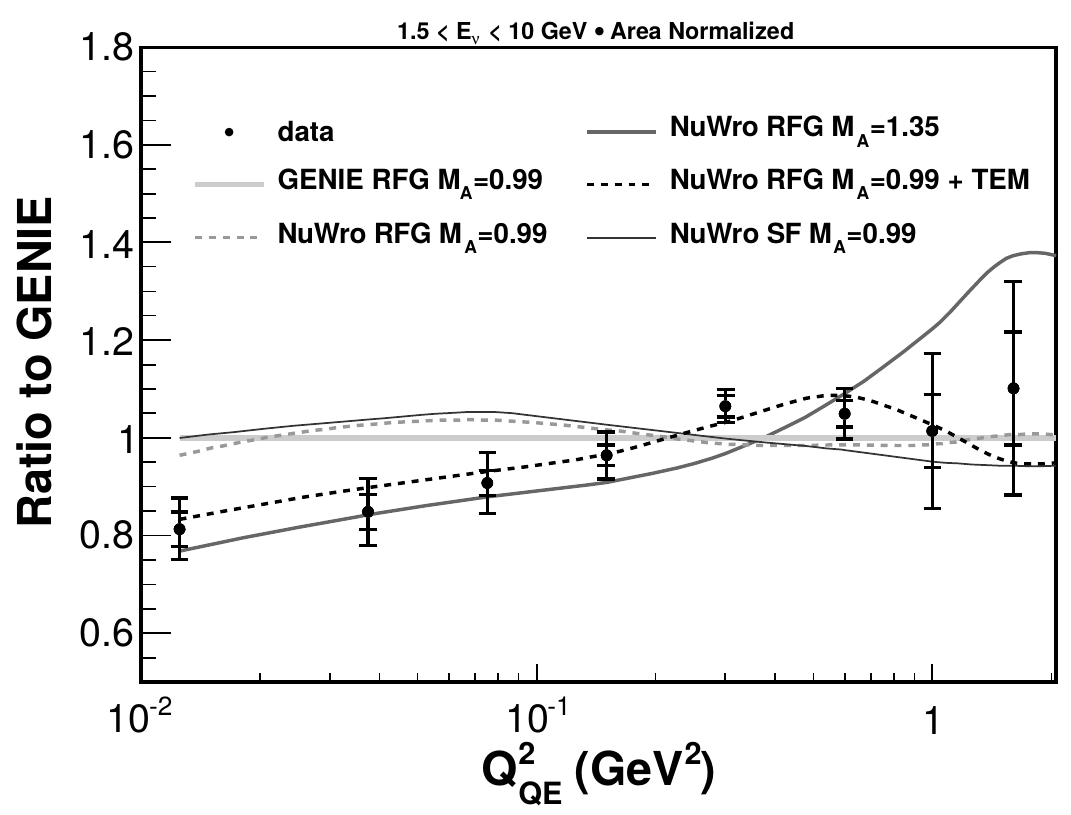## CCQE-"like" aka "muon+proton"

Flux Analysis Reference
Generation 1 $$\nu_\mu$$ muon + proton PRD (2015) 071301

• CC $$\nu_\mu$$ on $$CH$$

• require a muon, at least one proton, and no pions in the final state

• proton kinetic energy > 110 MeV

## $$\nu_\mu$$ CCQE-like## Charged pion production

CC $$1\pi^\pm$$

• require a muon and exactly one charged pion

• $$W < 1.4$$ GeV

CC $$N\pi^\pm$$

• require a muon and at least one charged pion

• $$W < 1.8$$ GeV

## Invariant mass

$E_\nu = E_\mu + E_{recoil}$ $Q^2 = 2E_\nu(E_\mu - |\vec p_\mu|\cos\theta_\mu) - m_\mu^2$ $W_{exp}^2 = M_p^2 - Q^2 + 2M_pE_{recoil}$

• $$E_{recoil}$$ is measured colorimetrically## Backrounds vs invariant mass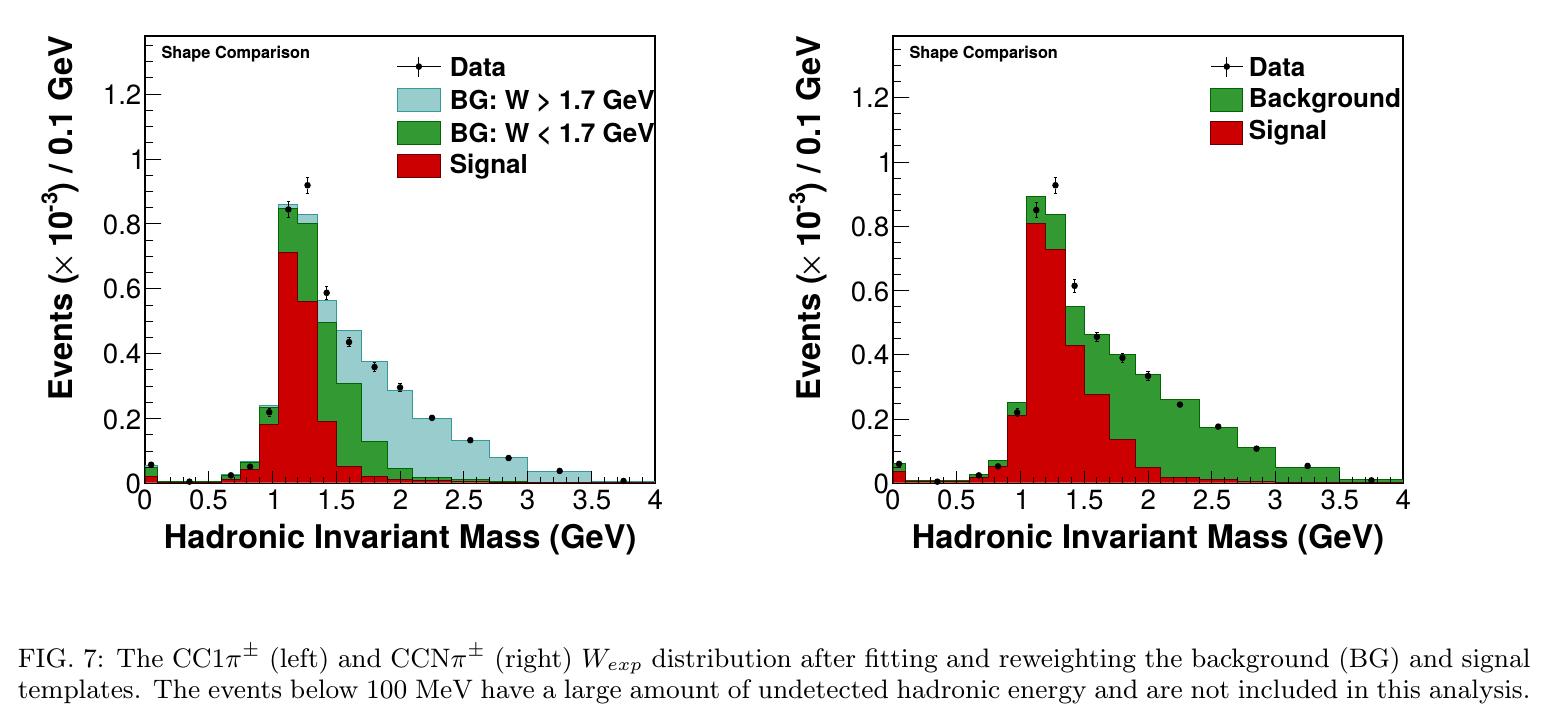## CC $$1\pi^\pm$$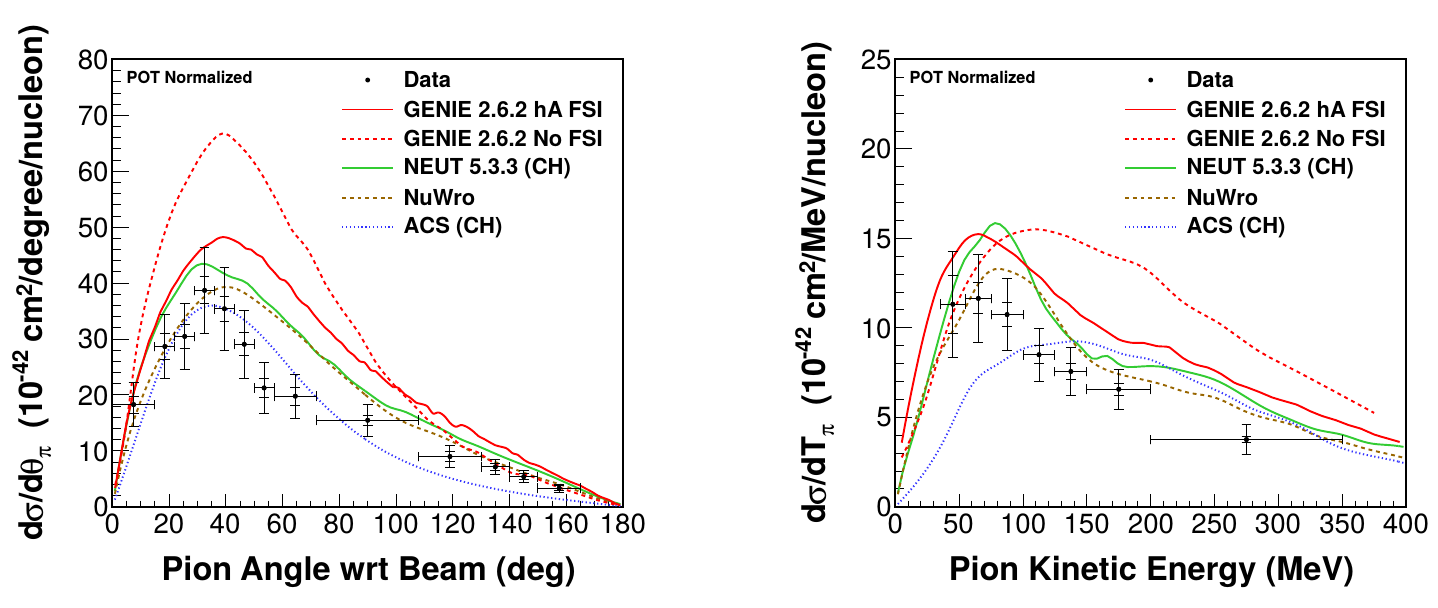## CC $$N\pi^\pm$$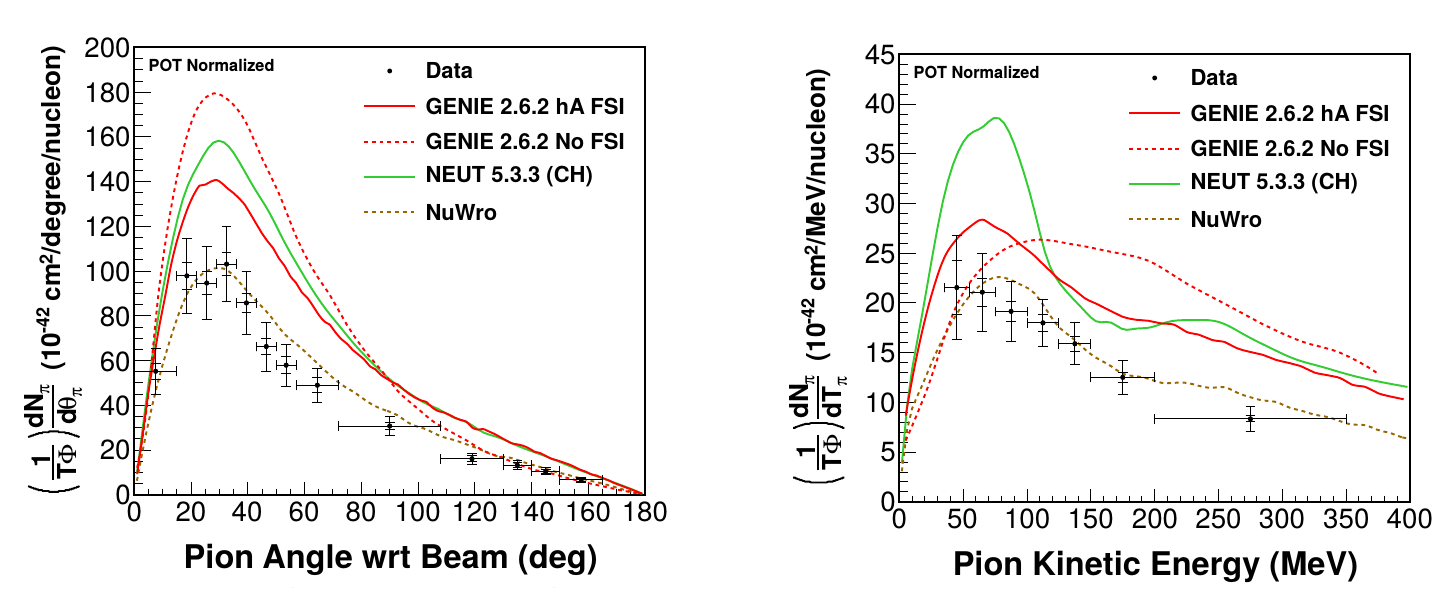## $$\bar\nu_\mu$$ CC $$1\pi^0$$

• require a muon and a single neutral pion (visible as two photons)
• about 70% of background - multipion events $$\pi^0 + \pi^\pm$$
• $$pi^\pm$$ is not tracked
• $$\pi^-\rightarrow\pi^0$$
• the rest of the background is mostly due to energy deposit by $$\pi^-$$ and neutrons misidentified as photons

## Invariant mass of $$\gamma\gamma$$

The $$\gamma\gamma$$ invariant mass is reconstructed from the photon energies ($$E_1$$, $$E_2$$):

$m_{\gamma\gamma}^2 = 2E_1E_2(1 - \cos\theta_{\gamma\gamma})$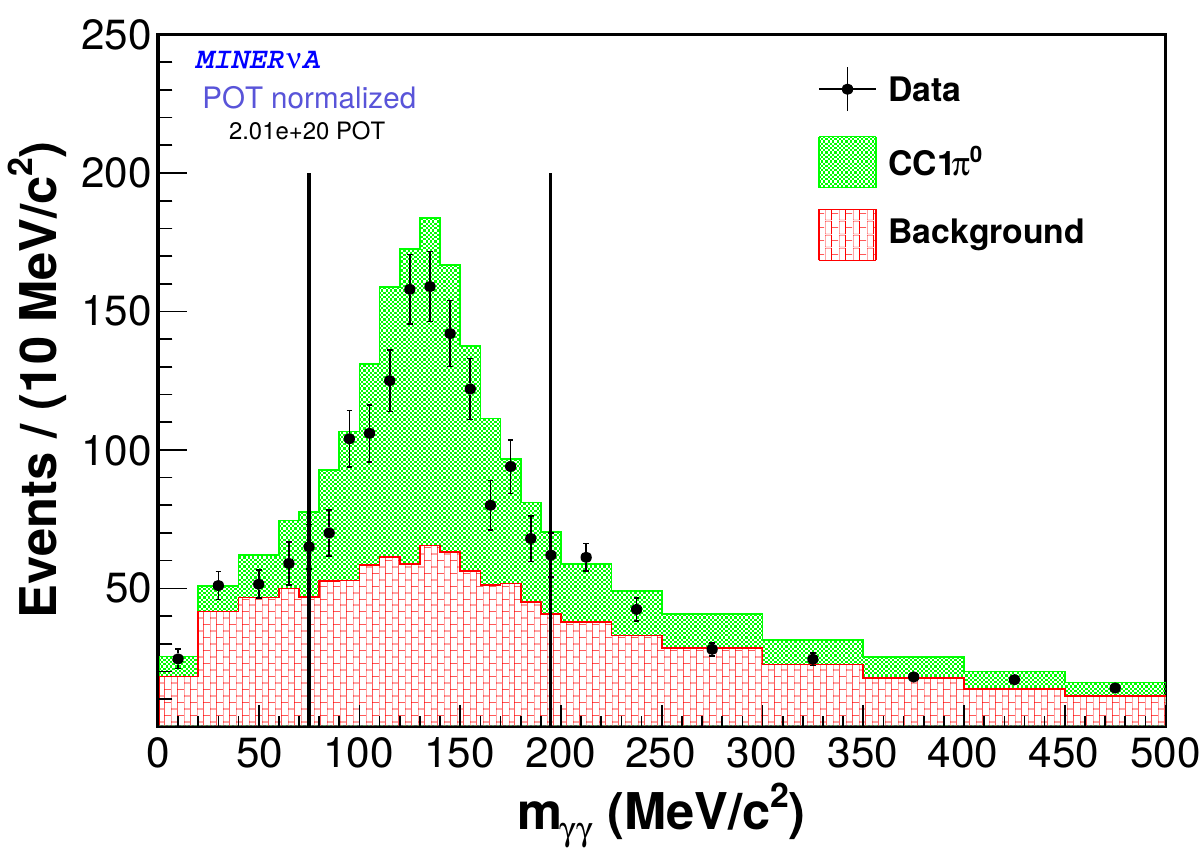## Differential cross sections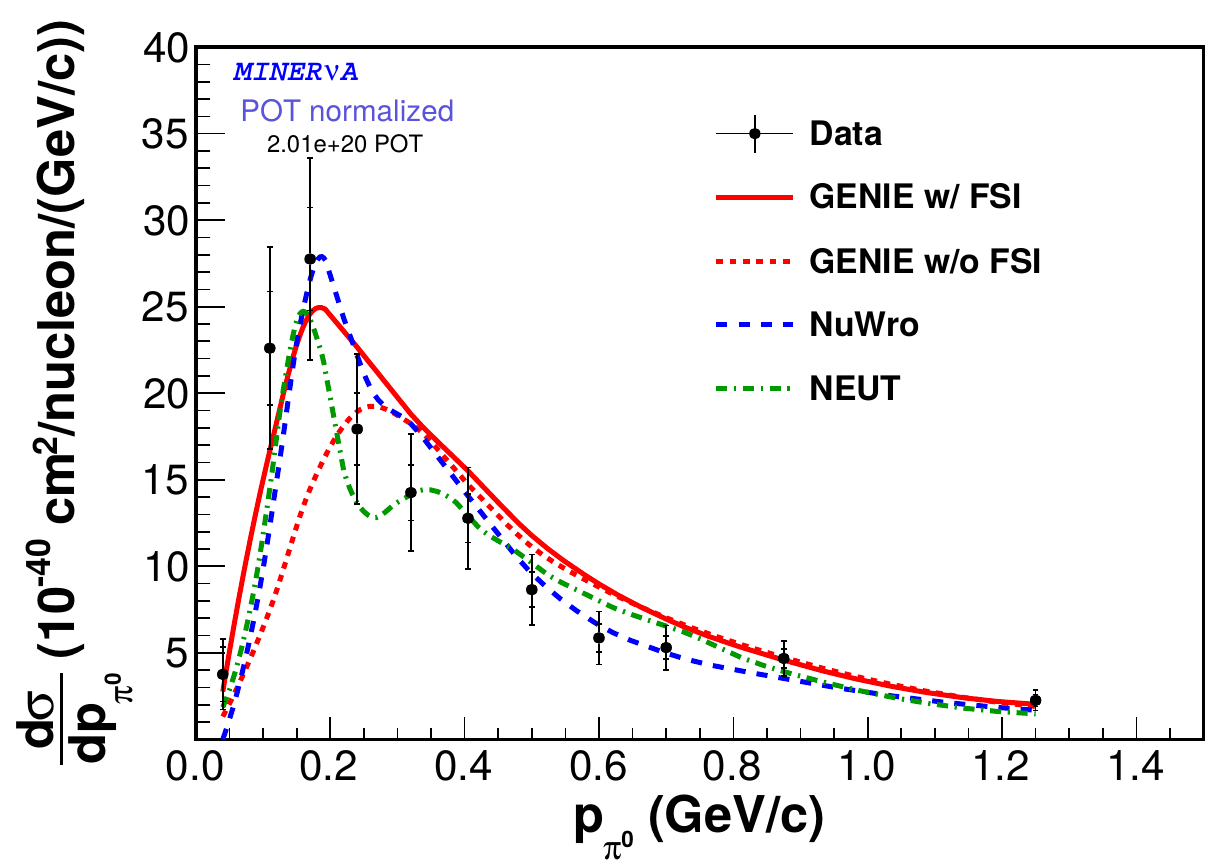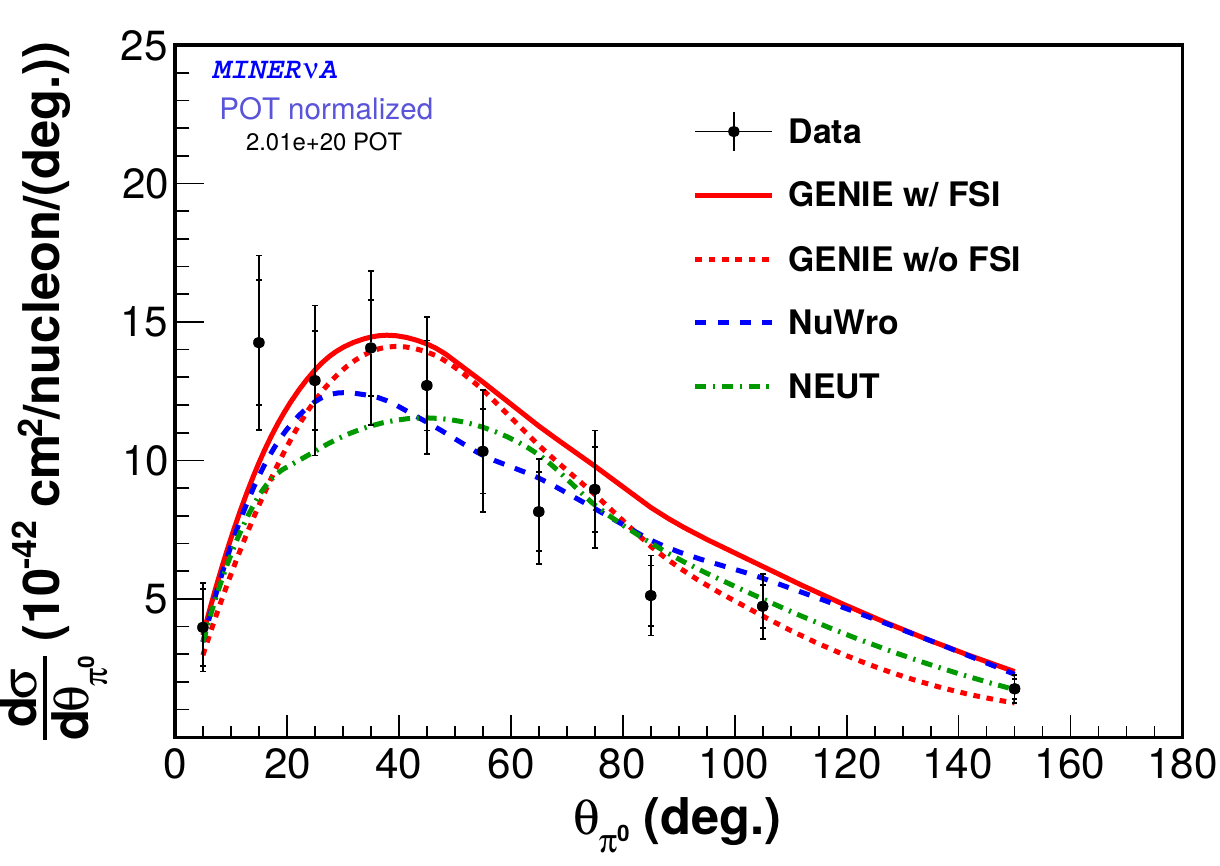## Coherent pion production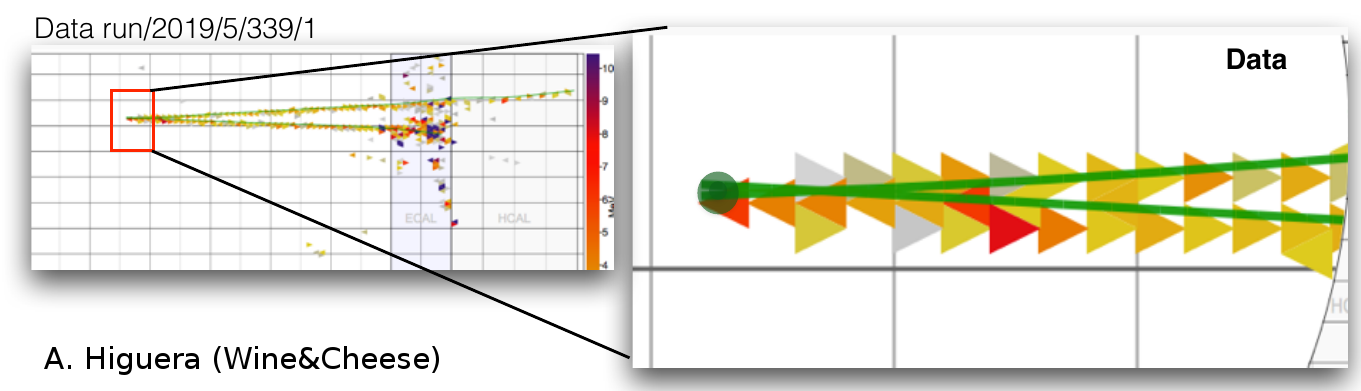• require two final state particles: $$\mu^\pm$$ and $$\pi^\mp$$ and no extra visible recoil

## Background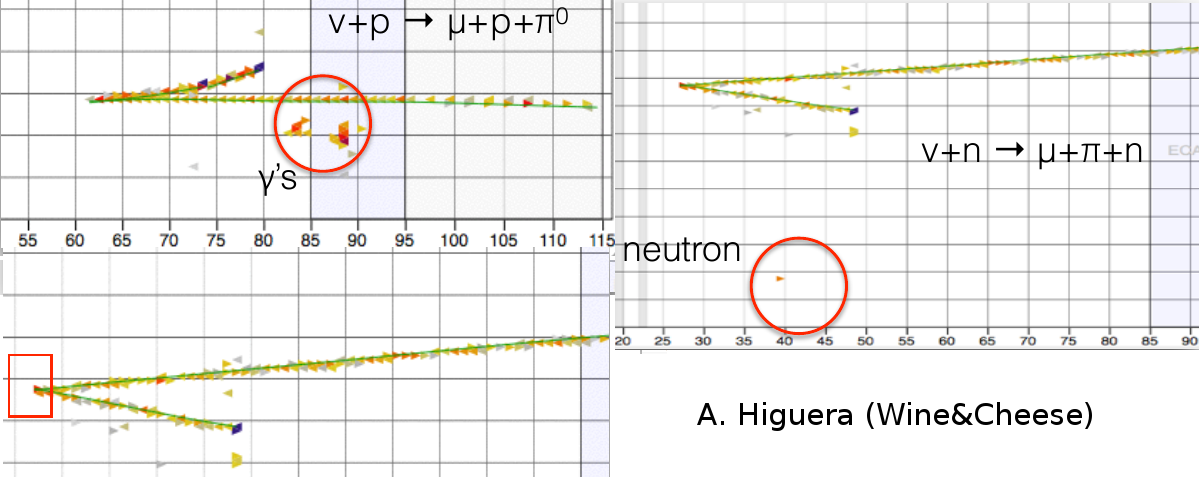## Low energy transfer requirement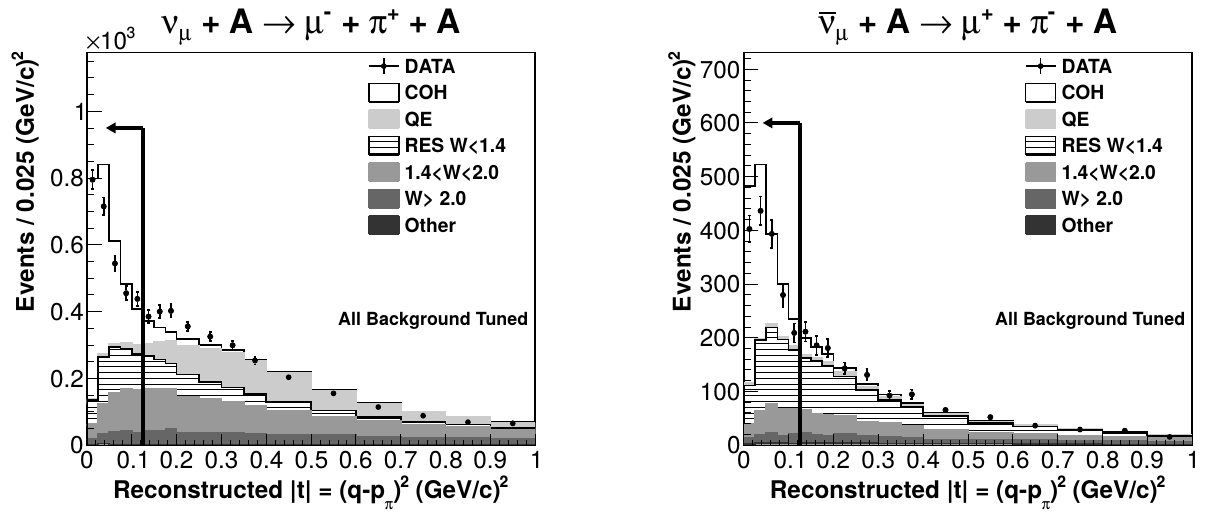## Total cross section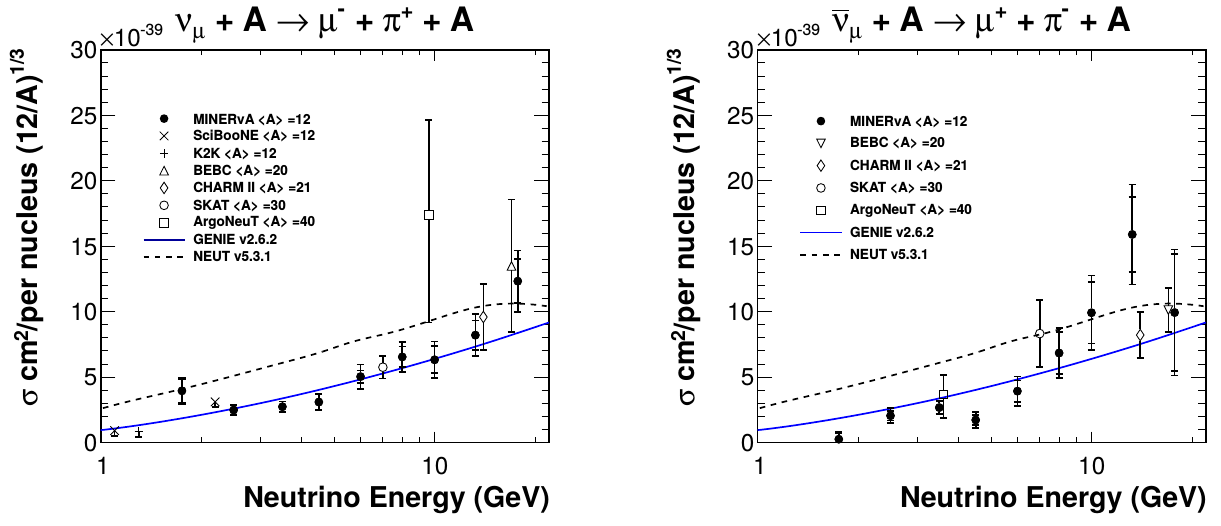## Differential cross section (energy)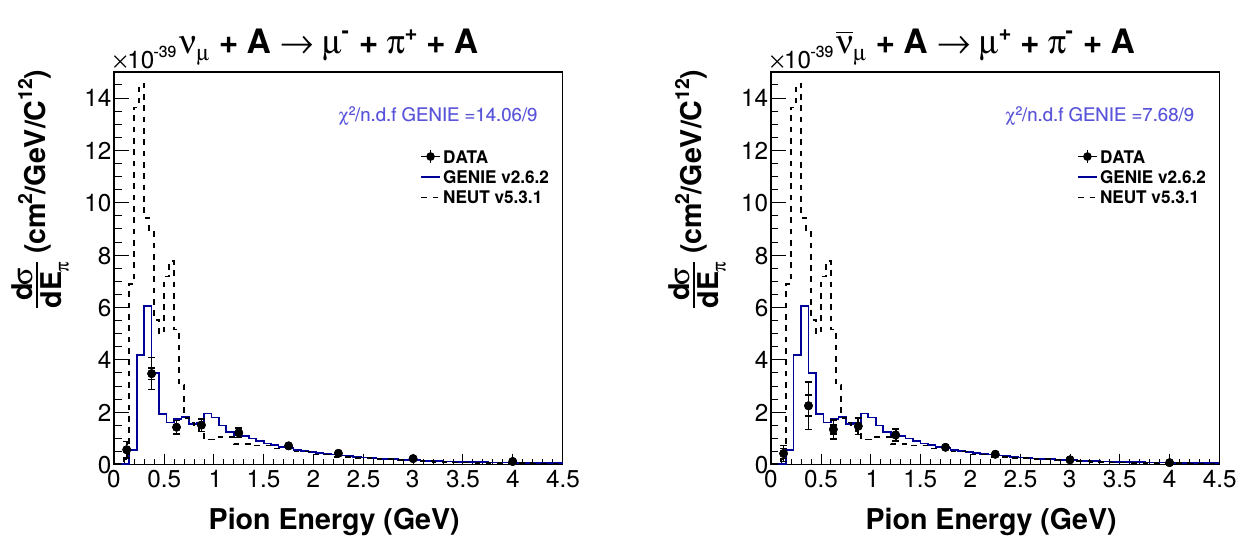## Differential cross section (angle)## Inclusive $$\nu_\mu$$ CC ratios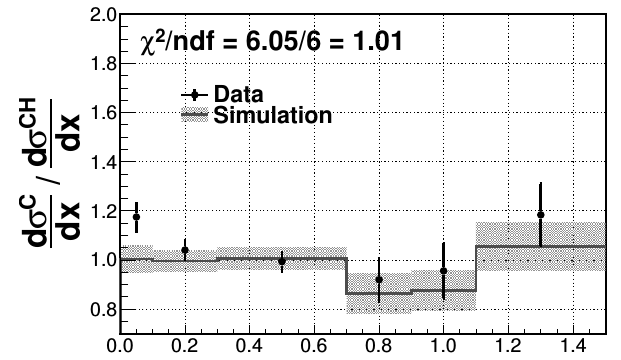• shadowing at low $$x$$

• no MEC in simulations (high $$x$$ dominated by QE)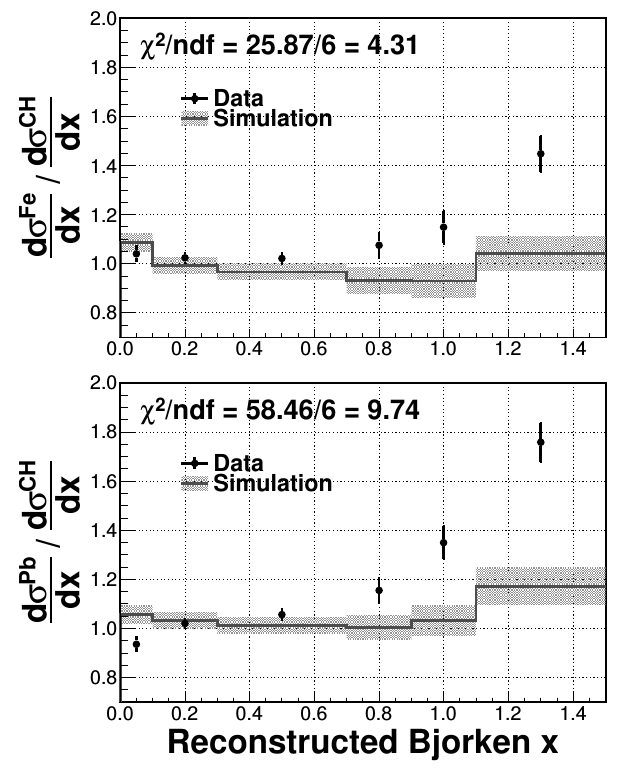## DIS $$\nu_\mu$$ CC ratios• $$W > 2$$ GeV and $$Q^2 > 1$$ GeV$$^2$$

• $$E_\nu$$ up to 50 GeV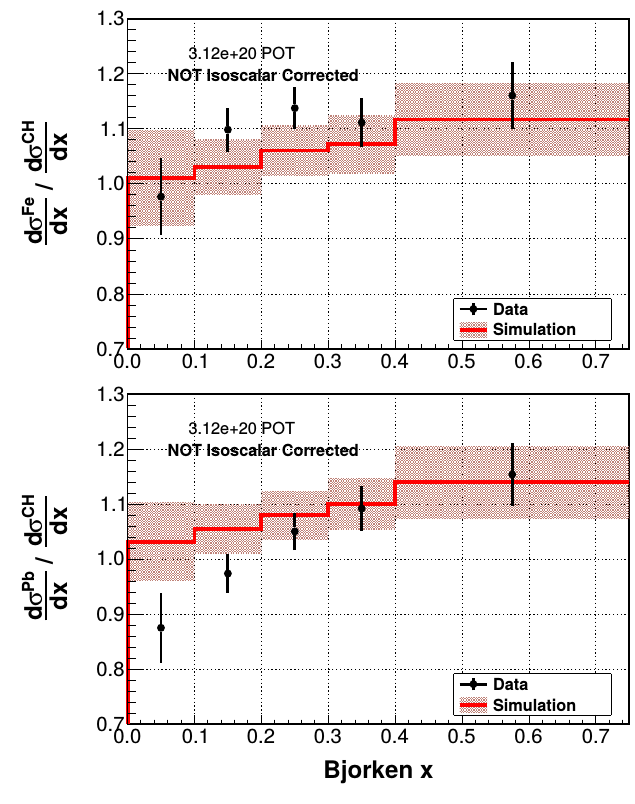## Available energy vs momentum transfer

• $$E_{avail}$$ - sum of proton and charged pion kinetic energy and neutral pion, electron, and photon total energy## NC diffractive $$\pi^0$$ production

• The most plausible source of the excess seen in the data is diffractive NC $$\pi^0$$ production from hydrogen in the scintillator target of MINERvA.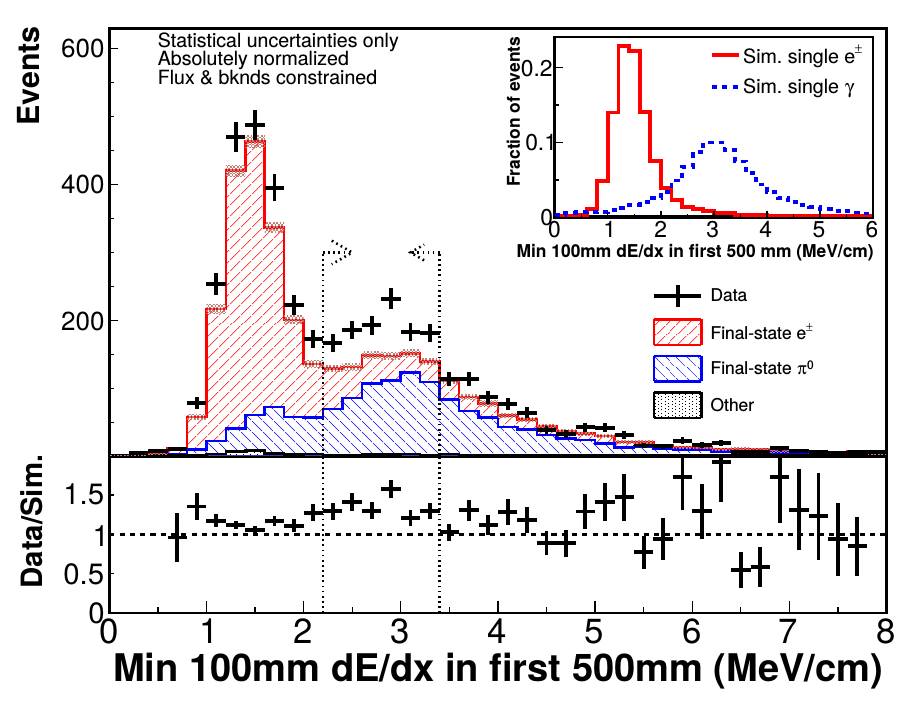## CC pion production (muon variables)

• $$\nu$$-CC($$\pi^+$$) and $$\bar\nu$$-CC($$\pi^0$$)

• total cross section

• differential cross sections:

• muon momentum
• muon angle
• $$Q^2$$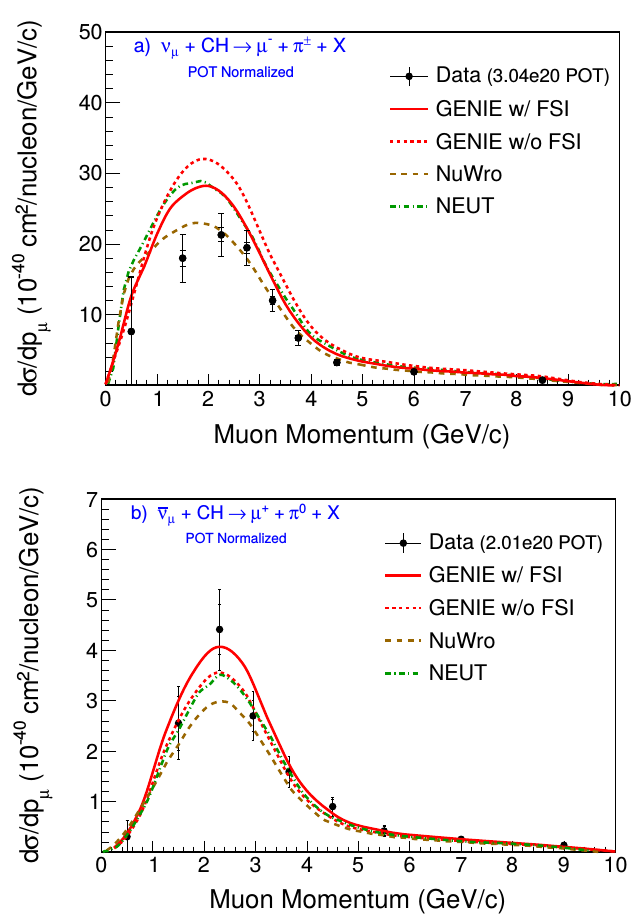## Kaon production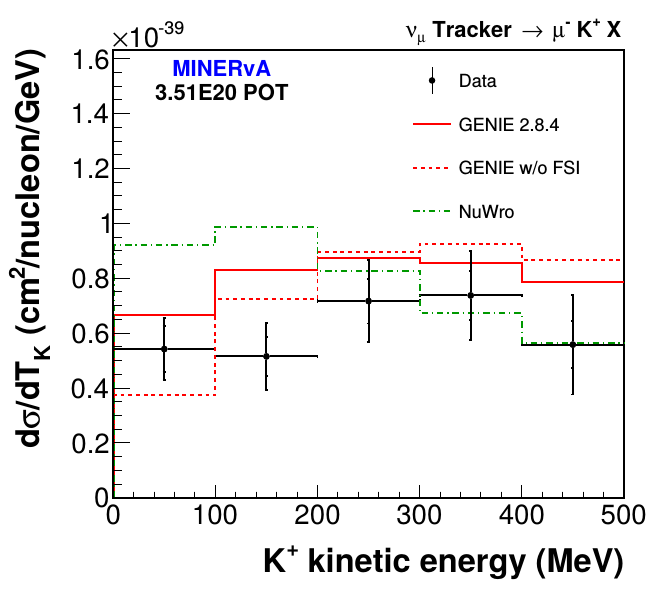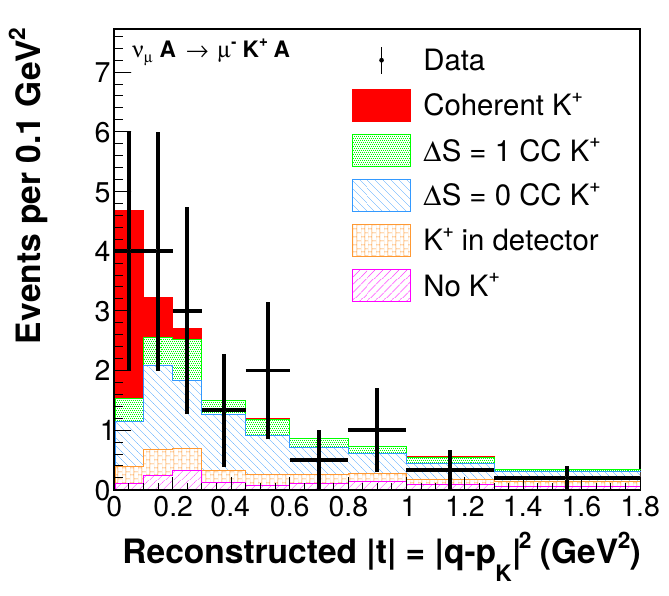## Summary

• MINERvA offers an unique opportunity to measure neutrino cross section on different nuclear targets

• There is still a lot of collected data to study (e.g. in nuclear target region)

• Medium energy data will allow to study more precisely DIS (and transition region?)